• 使用matlab编程实现的遗传算法，解决旅行商问题。。。。
• MATLAB语言编写tsp问题程序并仿真求解遍历34座城市最短路径。 1模拟退火首先从某个初始候选解开始，当温度大于0时执行循环。 2.在循环中通过随机扰动产生一个新的解，然后求得新解和原解之间的能量差，如果差小于0...
• 201801最近邻法与模拟退火算法求解TSP旅行商问题Matlab程序.pdf
• 禁忌搜索（TS）是基于本地搜索的元启发式方法，由Fred W. Glover于1986年提出。在本文中，我们将提供禁忌搜索（TS）算法求解旅行商问题（TSP）的matlab源代码
• 本代码实现了蚁群算法并且很好的解决了旅行商问题，对比了多个城市的结果，给出了最优路径图。
• 实现旅行商问题，采用matlab元工具箱自带工具包分析编程
• 遗传算法解决5种多旅行商问题（mtsp）的matlab程序 分别为以下5中情况： 1.从不同起点出发回到起点（固定旅行商数量） 2.从不同起点出发回到起点（旅行商数量根据计算可变） 3.从同一起点出发回到起点 4.从同一起点...
• MATLAB源码集锦-基于连续Hopfield神经网络的旅行商问题优化计算程序
• 旅行商问题动态规划matlab代码这是解决经典TSP的三种不同方法，即。 所有代码都在MATLAB 2019b上进行了测试。 算法是 遗传算法（边缘表示和2-opt） 动态编程 群算法（蚂蚁系统算法） 怎么跑 在遗传算法和群算法中，...
• 程序为经典的蚁群算法解决旅行商问题,蚁群算法包括信息素更新，蚂蚁数目，最大迭代次数等
• 经过计算机验证，很好用。 function varargout = mtsp_ga(xy,dmat,salesmen,min_tour,pop_size,num_iter,show_prog,show_res)mtsp
• 遗传算法解决5种多旅行商问题（mtsp）的matlab程序 从不同起点出发回到起点（固定旅行商数量） 从不同起点出发回到起点（旅行商数量根据计算可变） 从同一起点出发回到起点 从同一起点出发不会到起点
• 本资源用matlab实现了粒子群算法，并解决了旅行商问题。其中给出了TSP问题最优解的路径图以及收敛次数等信息。
• 数学建模中旅行商问题的遗传算法MATLAB程序，规划出合适的路径并展示出图形。
• 旅行商问题（动态规划方法，超级详细的） 在这就不细说了 直接看代码： 完整代码： function [circle,dis]=minCycle_dp(adjMat,pntSet,startPnt) %该函数使用动态规划法求解旅行商问题，点数不宜过多 %变量 类型 ...
具体实现方法可以参考这一篇呀： 旅行商问题（动态规划方法，超级详细的） 在这就不细说了
直接看代码： 完整代码：
function [circle,dis]=minCycle_dp(adjMat,pntSet,startPnt)

%该函数使用动态规划法求解旅行商问题，点数不宜过多
%变量        类型    　释义
%======================================================
%pntSet　 |  向量  |  各个点编号集合，无输入默认为1:N
%startPnt |  数值  |  圈起始点，无输入默认编号集的第一个
%------------------------------------------------------
%circle　 |  向量  |  路径最小圈
%dis      |  数值  |  最短路程

%实例：
%        3   0   3  10   5;
%       inf  3   0   4   3;
%        8  10   4   0  20;
%        9   5   3  20   0];
%
%                       邻接矩阵 编号
%                          ↓     ↓
%--------------------------
%实例运行结果：
%circle =
%    0  1  4  2  3  0
%dis =
%    23

%输入变量的初步处理、缺省参数赋值==========================================
switch 1
case nargin==1,pntSet=1:N;startPnt=1;
case nargin==2,startPnt=pntSet(1);
end
pntSet=pntSet(:);

%动态规划法程序主要部分：dp数组表格计算====================================
dpSheet=inf.*ones(N,2^(N-1));
for m=1:N
end
for m=1:2^(N-1)
for n=1:N
binNum=dec2bin(m-1);
binNum=binNum(length(binNum):-1:1);
comSet=find(binNum=='1');
for k=1:length(comSet)
subSet=comSet;
subSet(subSet==comSet(k))=[];
decNum=sum(2.^(subSet-1))+1;
end
end
end
end

%通过dp数组表格还原路径====================================================
circlePath=1;
comSet=2:N;
while ~isempty(comSet)
tempNum=1;
tempD=inf;
for m=1:length(comSet)
subSet=comSet;
subSet(subSet==comSet(m))=[];
decNum=sum(2.^(subSet-2))+1;
tempNum=comSet(m);
end
end
circlePath=[circlePath,tempNum];
comSet(comSet==tempNum)=[];
end

%为圈中节点赋予编号，改变圈起点，计算圈总长================================
circle=pntSet(circlePath)';
startPntPos=find(circle==startPnt);
circle=[circle(startPntPos:end),circle(1:startPntPos)];

end

. 使用实例：展开全文动态规划
• 遗传算法解决5种多旅行商问题（mtsp）的matlab程序 分别为以下5中情况： 1.从不同起点出发回到起点（固定旅行商数量） 2.从不同起点出发回到起点（旅行商数量根据计算可变） 3.从同一起点出发回到起点 4.从同一起点...
• 遗传算法解决5种多旅行商问题（mtsp）的matlab程序 分别为以下5中情况： 1.从不同起点出发回到起点（固定旅行商数量） 2.从不同起点出发回到起点（旅行商数量根据计算可变） 3.从同一起点出发回到起点 4.从同一起点...
• 文章目录前言一、TSP旅行商是什么？二、各种算法求解TSP问题1.遗传算法2.蚁群算法3....最早的旅行商问题的数学规划是由Dantzig（1959）等人提出，并且是在最优化领域中进行了深入研究。许多优化方法都用它


文章目录
前言一、TSP旅行商是什么？二、各种算法求解TSP问题1.遗传算法2.蚁群算法3.禁忌搜索算法4.模拟退火算法
总结

前言
旅行推销员问题（英语：Travelling salesman problem, TSP）是这样一个问题：给定一系列城市和每对城市之间的距离，求解访问每一座城市一次并回到起始城市的最短回路。它是组合优化中的一个NP难问题，在运筹学和理论计算机科学中非常重要。 最早的旅行商问题的数学规划是由Dantzig（1959）等人提出，并且是在最优化领域中进行了深入研究。许多优化方法都用它作为一个测试基准。尽管问题在计算上很困难，但已经有了大量的启发式算法和精确方法来求解数量上万的实例，并且能将误差控制在1%内。
一、TSP旅行商是什么？

旅行商问题(TravelingSalesmanProblem，TSP)是一个经典的组合优化问题。经典的TSP可以描述为：一个商品推销员要去若干个城市推销商品，该推销员从一个城市出发，需要经过所有城市后，回到出发地。应如何选择行进路线，以使总的行程最短。从图论的角度来看，该问题实质是在一个带权完全无向图中，找一个权值最小的Hamilton回路。由于该问题的可行解是所有顶点的全排列，随着顶点数的增加，会产生组合爆炸，它是一个NP完全问题。由于其在交通运输、电路板线路设计以及物流配送等领域内有着广泛的应用，国内外学者对其进行了大量的研究。早期的研究者使用精确算法求解该问题，常用的方法包括：分枝定界法、线性规划法、动态规划法等。但是，随着问题规模的增大，精确算法将变得无能为力，因此，在后来的研究中，国内外学者重点使用近似算法或启发式算法，主要有遗传算法、模拟退火法、蚁群算法、禁忌搜索算法、贪婪算法和神经网络等。
二、各种算法求解TSP问题
1.遗传算法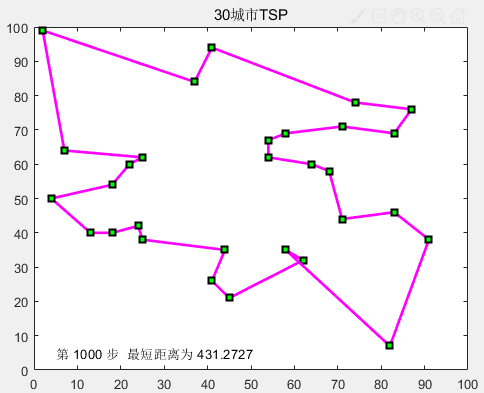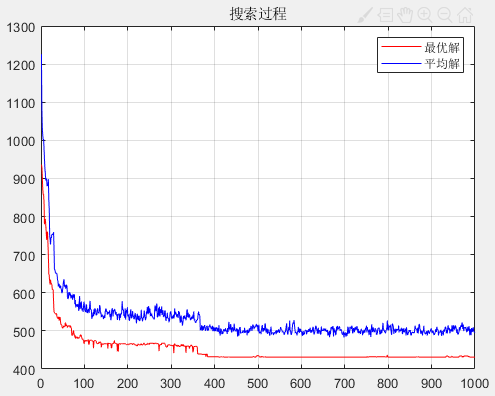2.蚁群算法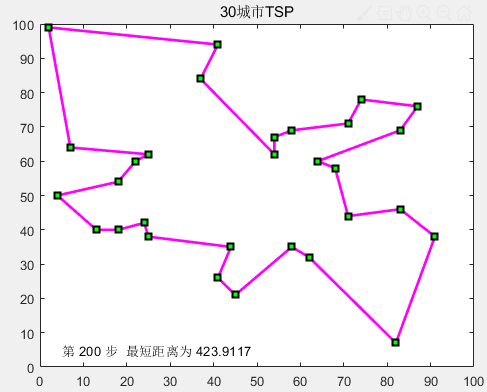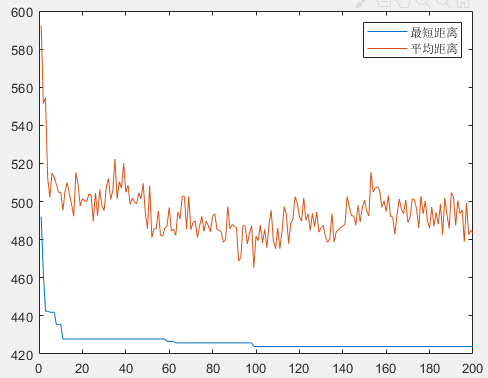3.禁忌搜索算法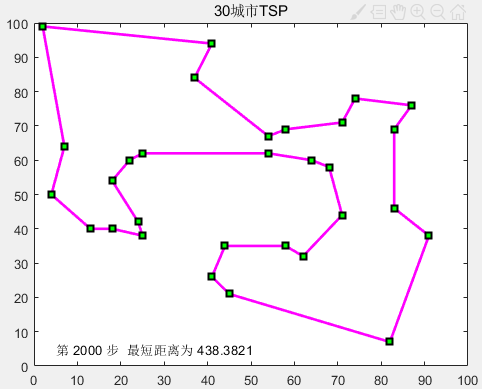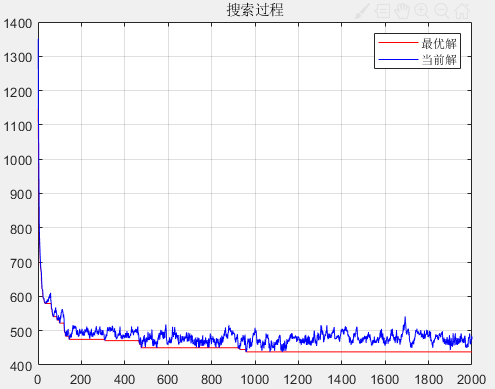4.模拟退火算法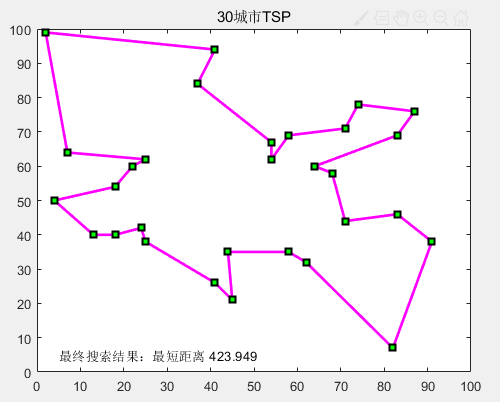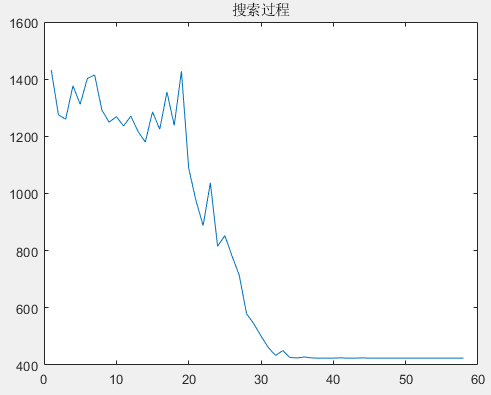总结
以上介绍了遗传算法、蚁群算法、禁忌搜索算法和模拟退火算法等对TSP旅行商问题的求解，希望有所帮助。

展开全文• 蚁群算法应用于旅行商问题的经典应用。程序MATLAB2018b编写完成，内附详尽注释。
• TSP_NN 旅行商问题 (TSP) 最近邻 (NN) 算法最近邻算法产生不同的结果取决于选择哪个城市作为起点。 这个函数决定多个起点和返回的最近邻路线这些路线中最好的概括： 1. 一名推销员前往每个城市并完成返回他出发的...
• 旅行商问题Matlab程序，数学建模竞赛时可能会用到
• 学习启发式算法时，旅行商问题是一个经典的例子。其中，遗传算法可以用来求解该问题。遗传算法是一种进化算法，由于其启发式算法的属性，并不能保证得到最优解。求解效果与初始种群选取，编码方法，选择方法，交叉...
学习启发式算法时，旅行商问题是一个经典的例子。其中，遗传算法可以用来求解该问题。遗传算法是一种进化算法，由于其启发式算法的属性，并不能保证得到最优解。求解效果与初始种群选取，编码方法，选择方法，交叉变异规则有关。
上课时，老师不知从哪里找了一个非常粗糙的程序，自己将不少错误修正，增加了一些注释方便理解，并增加了一些代码使程序更完美。该代码可以动态显示每一代的路线，非常直观！ （备注：对于一般的 TSP 问题，发现通过将其构造成整数规划问题，利用数学规划求解软件 Gurobi 中的 Callback 方法求解，即使大规模问题 （75 个城市）也可以在 1 秒钟内得到最优解。就没必要用启发式算法了。参看网址：https://www.gurobi.com/resource/traveling-salesman-problem/ ）
本程序的显示效果：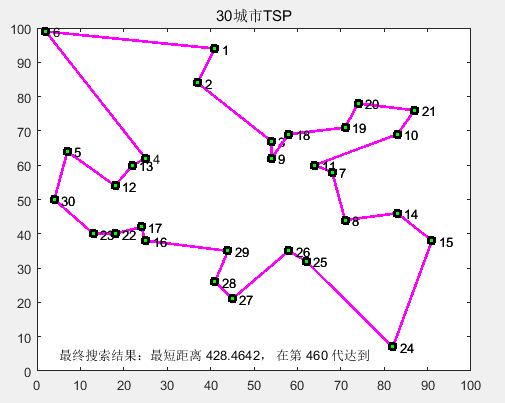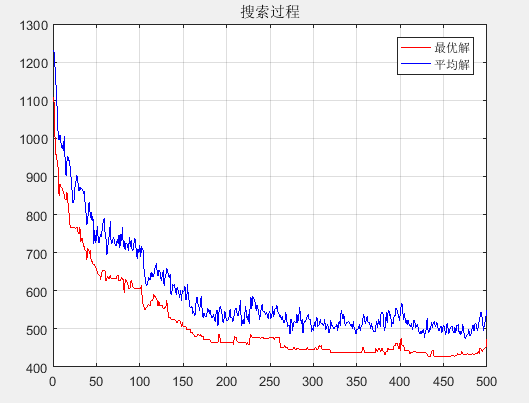代码：
function GaTSPChen

% mainly amended by Chen Zhen, 2012~2016

CityNum = 30; % 城市数目，可以选 10, 30, 50, 75
[dislist, Clist] = tsp(CityNum); % dislist 为城市之间相互的距离，Clist 为各城市的坐标

inn = 30; % 初始种群大小
gnMax = 500;  % 最大代数
crossProb = 0.8; % 交叉概率
muteProb = 0.8; % 变异概率

% 随机产生初始种群
population = zeros(inn, CityNum); % population 为初始种群，包括多条染色体
for i = 1 : inn
population(i,:) = randperm(CityNum);
end
[~, cumulativeProbs] = calPopulationValue(population, dislist); % 计算种群每条染色体的累计概率

generationNum = 1;
generationMeanValue = zeros(generationNum, 1); % 每一代的平均距离
generationMaxValue = zeros(generationNum, 1);  % 每一代的最短距离
bestRoute = zeros(inn, CityNum); % 最佳路径
newPopulation = zeros(inn, CityNum); % 新的种群
while generationNum < gnMax + 1
for j = 1 : 2 : inn
selectedChromos = select(cumulativeProbs);  % 选择操作，选出两条需要交叉编译的染色体，即父亲母亲
crossedChromos = cross(population, selectedChromos, crossProb);  % 交叉操作，返回交叉后的染色体
newPopulation(j, :) = mut(crossedChromos(1, :),muteProb);  % 对交叉后的染色体进行变异操作
newPopulation(j + 1, :) = mut(crossedChromos(2, :), muteProb); % 对交叉后的染色体进行变异操作
end
population = newPopulation;  %产生了新的种群
[populationValue, cumulativeProbs] = calPopulationValue(population, dislist);  % 计算新种群的适应度
% 记录当前代最好和平均的适应度
[fmax, nmax] = max(populationValue); % 因为计算适应度时取距离的倒数，这里面取最大的倒数，即最短的距离
generationMeanValue(generationNum) = 1 / mean(populationValue);
generationMaxValue(generationNum) = 1 / fmax;
bestChromo = population(nmax, :);  % 前代最佳染色体，即对应的路径
bestRoute(generationNum, :) = bestChromo; % 记录每一代的最佳染色体
drawTSP(Clist, bestChromo, generationMaxValue(generationNum), generationNum, 0);
generationNum = generationNum + 1;
end
[bestValue,index] = min(generationMaxValue);
drawTSP(Clist, bestRoute(index, :), bestValue, index,1);

figure(2);
plot(generationMaxValue, 'r');
hold on;
plot(generationMeanValue, 'b');
grid;
title('搜索过程');
legend('最优解', '平均解');
fprintf('遗传算法得到的最短距离: %.2f\n', bestValue);
fprintf('遗传算法得到的最短路线');
disp(bestRoute(index, :));
end

%------------------------------------------------
% 计算所有染色体的适应度
function [chromoValues, cumulativeProbs] = calPopulationValue(s, dislist)
inn = size(s, 1);  % 读取种群大小
chromoValues = zeros(inn, 1);
for i = 1 : inn
chromoValues(i) = CalDist(dislist, s(i, :));  % 计算每条染色体的适应度
end
chromoValues = 1./chromoValues'; % 因为让距离越小，选取的概率越高，所以取距离倒数

% 根据个体的适应度计算其被选择的概率
fsum = 0;
for i = 1 : inn
% 乘以15次方的原因是让好的个体被选取的概率更大（因为适应度取距离的倒数，若不乘次方，则个体相互之间的适应度差别不大），换成一个较大的数也行
fsum = fsum + chromoValues(i)^15;
end

% 计算单个概率
probs = zeros(inn, 1);
for i = 1: inn
probs(i) = chromoValues(i)^15 / fsum;
end

% 计算累积概率
cumulativeProbs = zeros(inn,1);
cumulativeProbs(1) = probs(1);
for i = 2 : inn
cumulativeProbs(i) = cumulativeProbs(i - 1) + probs(i);
end
cumulativeProbs = cumulativeProbs';
end

%--------------------------------------------------
%“选择”操作，返回所选择染色体在种群中对应的位置
% cumulatedPro 所有染色体的累计概率
function selectedChromoNums = select(cumulatedPro)
selectedChromoNums = zeros(2, 1);
% 从种群中选择两个个体，最好不要两次选择同一个个体
for i = 1 : 2
r = rand;  % 产生一个随机数
prand = cumulatedPro - r;
j = 1;
while prand(j) < 0
j = j + 1;
end
selectedChromoNums(i) = j; % 选中个体的序号
if i == 2 && j == selectedChromoNums(i - 1)    % 若相同就再选一次
r = rand;  % 产生一个随机数
prand = cumulatedPro - r;
j = 1;
while prand(j) < 0
j = j + 1;
end
end
end
end

%------------------------------------------------
% “交叉”操作
function crossedChromos = cross(population, selectedChromoNums, crossProb)
length = size(population, 2); % 染色体的长度
crossProbc = crossMuteOrNot(crossProb);  %根据交叉概率决定是否进行交叉操作，1则是，0则否
crossedChromos(1,:) = population(selectedChromoNums(1), :);
crossedChromos(2,:) = population(selectedChromoNums(2), :);
if crossProbc == 1
c1 = round(rand * (length - 2)) + 1;  %在[1,bn - 1]范围内随机产生一个交叉位 c1
c2 = round(rand * (length - 2)) + 1;  %在[1,bn - 1]范围内随机产生一个交叉位 c2
chb1 = min(c1, c2);
chb2 = max(c1,c2);
middle = crossedChromos(1,chb1+1:chb2); % 两条染色体 chb1 到 chb2 之间互换位置
crossedChromos(1,chb1 + 1 : chb2)= crossedChromos(2, chb1 + 1 : chb2);
crossedChromos(2,chb1 + 1 : chb2)= middle;
for i = 1 : chb1 % 看交叉后，染色体上是否有相同编码的情况（路径上重复出现两个城市）。若有，则该编码不参与交叉
while find(crossedChromos(1,chb1 + 1: chb2) == crossedChromos(1, i))
location = find(crossedChromos(1,chb1 + 1: chb2) == crossedChromos(1, i));
y = crossedChromos(2,chb1 + location);
crossedChromos(1, i) = y;
end
while find(crossedChromos(2,chb1 + 1 : chb2) == crossedChromos(2, i))
location = find(crossedChromos(2, chb1 + 1 : chb2) == crossedChromos(2, i));
y = crossedChromos(1, chb1 + location);
crossedChromos(2, i) = y;
end
end
for i = chb2 + 1 : length
while find(crossedChromos(1, 1 : chb2) == crossedChromos(1, i))
location = logical(crossedChromos(1, 1 : chb2) == crossedChromos(1, i));
y = crossedChromos(2, location);
crossedChromos(1, i) = y;
end
while find(crossedChromos(2, 1 : chb2) == crossedChromos(2, i))
location = logical(crossedChromos(2, 1 : chb2) == crossedChromos(2, i));
y = crossedChromos(1, location);
crossedChromos(2, i) = y;
end
end
end
end

%--------------------------------------------------
%“变异”操作
% choromo 为一条染色体
function snnew = mut(chromo,muteProb)
length = size(chromo, 2); % 染色体的的长度
snnew = chromo;
muteProbm = crossMuteOrNot(muteProb);  % 根据变异概率决定是否进行变异操作，1则是，0则否
if muteProbm == 1
c1 = round(rand*(length - 2)) + 1;  % 在 [1, bn - 1]范围内随机产生一个变异位
c2 = round(rand*(length - 2)) + 1;  % 在 [1, bn - 1]范围内随机产生一个变异位
chb1 = min(c1, c2);
chb2 = max(c1, c2);
x = chromo(chb1 + 1 : chb2);
snnew(chb1 + 1 : chb2) = fliplr(x); % 变异，则将两个变异位置的染色体倒转
end
end

% 根据变异或交叉概率，返回一个 0 或 1 的数
function crossProbc = crossMuteOrNot(crossMuteProb)
test(1: 100) = 0;
l = round(100 * crossMuteProb);
test(1 : l) = 1;
n = round(rand * 99) + 1;
crossProbc = test(n);
end

%------------------------------------------------
% 计算一条染色体的适应度
% dislist 为所有城市相互之间的距离矩阵
% chromo 为一条染色体，即一条路径
function chromoValue = CalDist(dislist, chromo)
DistanV = 0;
n = size(chromo, 2); % 染色体的长度
for i = 1 : (n - 1)
DistanV = DistanV + dislist(chromo(i), chromo(i + 1));
end
DistanV = DistanV + dislist(chromo(n), chromo(1));
chromoValue = DistanV;
end

%------------------------------------------------
% 画图
% Clist 为城市坐标
% route 为一条路径
function drawTSP(Clist, route, generationValue, generationNum,isBestGeneration)
CityNum = size(Clist, 1);
for i = 1 : CityNum - 1
plot([Clist(route(i), 1),Clist(route(i + 1), 1)], [Clist(route(i),2),Clist(route(i+1),2)],'ms-','LineWidth',2,'MarkerEdgeColor','k','MarkerFaceColor','g');
text(Clist(route(i), 1),Clist(route(i), 2), ['  ', int2str(route(i))]);
text(Clist(route(i+1), 1),Clist(route(i + 1), 2), ['  ', int2str(route(i+1))]);
hold on;
end
plot([Clist(route(CityNum), 1), Clist(route(1), 1)], [Clist(route(CityNum), 2), Clist(route(1), 2)],'ms-','LineWidth',2,'MarkerEdgeColor','k','MarkerFaceColor','g');
title([num2str(CityNum),'城市TSP']);
if isBestGeneration == 0 && CityNum ~= 10
text(5, 5, ['第 ',int2str(generationNum),' 代','  最短距离为 ', num2str(generationValue)]);
else
text(5, 5, ['最终搜索结果：最短距离 ',num2str(generationValue),'， 在第 ',num2str(generationNum),' 代达到']);
end
if CityNum == 10  % 因为文字显示位置不一样，所以将城市数目为 10 时单独编写
if isBestGeneration == 0
text(0, 0, ['第 ',int2str(generationNum),' 代','  最短距离为 ', num2str(generationValue)]);
else
text(0, 0, ['最终搜索结果：最短距离 ',num2str(generationValue),'， 在第 ', num2str(generationNum),' 代达到']);
end
end
hold off;
pause(0.005);
end

%------------------------------------------------
%城市位置坐标
function [DLn, cityn] = tsp(n)
DLn = zeros(n, n);
if n == 10
city10 = [0.4 0.4439;0.2439 0.1463;0.1707 0.2293;0.2293 0.761;0.5171 0.9414;
0.8732 0.6536;0.6878 0.5219;0.8488 0.3609;0.6683 0.2536;0.6195 0.2634];%10 cities d'=2.691
for i = 1 : 10
for j = 1 : 10
DLn(i, j) = ((city10(i,1)-city10(j,1))^2 + (city10(i,2)-city10(j,2))^2)^0.5;
end
end
cityn = city10;
end
if n == 30
city30 = [41 94;37 84;54 67;25 62;7 64;2 99;68 58;71 44;54 62;83 69;64 60;18 54;22 60;
83 46;91 38;25 38;24 42;58 69;71 71;74 78;87 76;18 40;13 40;82 7;62 32;58 35;45 21;41 26;44 35;4 50]; % 30 cities d' = 423.741 by D B Fogel
for i = 1 : 30
for j = 1 : 30
DLn(i,j) = ((city30(i,1)-city30(j,1))^2+(city30(i,2)-city30(j,2))^2)^0.5;
end
end
cityn = city30;
end

if n == 50
city50 = [31 32;32 39;40 30;37 69;27 68;37 52;38 46;31 62;30 48;21 47;25 55;16 57;
17 63;42 41;17 33;25 32;5 64;8 52;12 42;7 38;5 25; 10 77;45 35;42 57;32 22;
27 23;56 37;52 41;49 49;58 48;57 58;39 10;46 10;59 15;51 21;48 28;52 33;
58 27;61 33;62 63;20 26;5 6;13 13;21 10;30 15;36 16;62 42;63 69;52 64;43 67];%50 cities d'=427.855 by D B Fogel
for i = 1 : 50
for j = 1:50
DLn(i, j) = ((city50(i,1) - city50(j,1))^2 + (city50(i,2) - city50(j,2))^2)^0.5;
end
end
cityn = city50;
end

if n == 75
city75 = [48 21;52 26;55 50;50 50;41 46;51 42;55 45;38 33;33 34;45 35;40 37;50 30;
55 34;54 38;26 13;15 5;21 48;29 39;33 44;15 19;16 19;12 17;50 40;22 53;21 36;
20 30;26 29;40 20;36 26;62 48;67 41;62 35;65 27;62 24;55 20;35 51;30 50;
45 42;21 45;36 6;6 25;11 28;26 59;30 60;22 22;27 24;30 20;35 16;54 10;50 15;
44 13;35 60;40 60;40 66;31 76;47 66;50 70;57 72;55 65;2 38;7 43;9 56;15 56;
10 70;17 64;55 57;62 57;70 64;64 4;59 5;50 4;60 15;66 14;66 8;43 26]; % 75 cities d'=549.18 by D B Fogel
for i = 1 : 75
for j = 1 : 75
DLn(i,j) = ((city75(i,1)-city75(j,1))^2 + (city75(i,2)-city75(j,2))^2)^0.5;
end
end
cityn = city75;
end
end



展开全文遗传算法
• 5种多旅行商问题MATLAB实现方法 1.从不同起点出发回到起点（固定旅行商数量） 2.从同一起点出发回到起点 3.从同一起点出发回到同一终点（与起点不同） 4.从同一起点出发不回到起点 5.从不同起点出发回到起点...MTSP...

# 旅行商问题matlab程序matlab 订阅